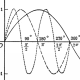﻿﻿ Introducing the multi-zeta function! – futurebird

# Introducing the multi-zeta function!

Contemplating:$\frac{\pi ^{2n}}{(2n+1)!} = \displaystyle \sum_{j_1,...j_n=1 \atop j_i \neq j_k}^{\infty} (j_1j_2...j_n)^{-2}$

My friend, and fellow math blogger, Owen, tried to tell me I was dealing with the multi-zeta function the other day, but the very general definition on WolframMathworld left me feeling a little mystified. I could see how the identities I was playing with fit in to it, but what was all of that other stuff (like$\sigma_1,...,\sigma_k$? I guess in my equation they are all 1? Why isn't it dealing with powers of the active variable in the product in the denominator? Why the extra level of subscripts?) So, I asked about it on stackexchange to gain more insight, and, thanks to Marni, I was promptly pointed to this lovely paper:

Now, I can see easily that the function is:$A(i_1, i_2, ... , i_k) = \sum_{n_1 > n_2 > \cdots > n_k > 1} \frac{1}{n_1^{i_1}n_2^{i_2} \cdots n_k^{i_k}}$

With$i_1, i_2, ... , i_k = 2$.

More on this later.

Meaning one may repeat values,futurebird

1.Owen Maresh says:
2.John Robinhūdas says: